Select Page

# Sequential Circuits – II

Sequential Circuits – II

Contents

Define and classify Counters 2

Construct Two-bit Ripple Up-Counter and Down Counter 3

Construct Two-bit Ripple Up/Down Counter 4

Design Mod-6 Asynchronous Counter 5

Design Mod-10 Asynchronous Counter 6

Recall the Effect of Propagation Delay in Ripple Counters 7

Design Ring Counter 8

Design Johnson Counter 9

Design Synchronous BCD Counter 11

Design 3-bit Synchronous Up-Counter 13

Design 3-bit Synchronous Down-Counter 16

Design 3-bit Synchronous Up/Down Counter 18

Design MOD-6 Synchronous Counter 20

Design MOD-9 Synchronous Counter 23

Recall Finite State Machine 25

Describe: 1. Moore Machine 2. Mealy Machine 26

Describe Moore Machine 29

Describe Mealy Machine 30

Describe Minimization of Sequential Machine 32

Recall State Reduction Methods: Successive Partitioning 36

Recall State Reduction Methods: Implication Chart 36

Describe Method of State Assignment 37

Recall Hazards and Its types 38

Differentiate between Static and Dynamic Hazards 39

Determine Hazards in Combinational Circuits 39

Recall Faults and its types 40

Differentiate between Struck-At and Bridging Faults 41

Differentiate between Single Stuck-At and Multiple Stuck-At Faults 41

Describe Faults Detection in Combinational and Sequential circuits 42

# Define and classify Counters

Counters are digital circuits that are used to count the number of events that occur over time. They are commonly used in applications such as frequency measurement, timekeeping, and control systems.

Counters can be classified into several categories based on their characteristics:

1. Asynchronous or Ripple Counters: These are the simplest type of counters and use flip-flops that are triggered by the output of the preceding stage. The output of each stage serves as a clock input to the next stage, resulting in a ripple effect as the count progresses. Asynchronous counters are simple to implement but can be slow and prone to glitches.
2. Synchronous Counters: These counters use a common clock signal to trigger all stages simultaneously. This results in a faster and more reliable count than asynchronous counters, but they require more complex circuitry.
3. Up Counters: These counters count up from a starting value, typically zero. They are the most common type of counter and can be implemented using either asynchronous or synchronous techniques.
4. Down Counters: These counters count down from a starting value, typically a preset value. They can also be implemented using either asynchronous or synchronous techniques.
5. Bidirectional Counters: These counters can count up or down based on a control signal. They are commonly used in applications where the count needs to change direction based on some external condition.
6. Ring Counters: These counters use a circular shift register to produce a sequence of output states. The count is determined by the number of stages in the shift register.
7. Johnson Counters: These counters are a variation of the ring counter, where the output sequence is complemented at each stage. This results in a sequence that repeats after 2n-1 states, where n is the number of stages in the counter.

Counters can also be classified based on their applications. For example, event counters count the number of events that occur in a system, while frequency counters measure the frequency of a signal. Time interval counters measure the time between two events, and programmable counters can be configured to count in different ways based on the input configuration.

Overall, counters are a fundamental building block in digital electronics, and their wide range of applications and configurations make them essential for many different types of digital systems.

# Construct Two-bit Ripple Up-Counter and Down Counter

Sure, here are the designs for a 2-bit ripple up-counter and down counter using D flip-flops: Two-bit Ripple Up-Counter:

The truth table for a 2-bit ripple up-counter is:

 Q1 Q0 Count 0 0 0 0 1 1 1 0 2 1 1 3

# Construct Two-bit Ripple Up/Down Counter

Sure, here’s the design for a 2-bit ripple up/down counter using D flip-flops:

Two-bit Ripple Up/Down Counter:

The truth table for a 2-bit ripple up/down counter is:

 Q1 Q0 Count 0 0 0 0 1 1 1 0 2 1 1 3

# Design Mod-6 Asynchronous Counter

Sure, here’s the design for a mod-6 asynchronous counter using J-K flip-flops:

Mod-6 Asynchronous Counter:

The truth table for a mod-6 asynchronous counter is:

 Q2 Q1 Q0 Count 0 0 0 0 0 0 1 1 0 1 0 2

# Design Mod-10 Asynchronous Counter

A Mod-10 Asynchronous Counter can be designed using a combination of a Mod-2 counter and a Mod-5 counter. The Mod-2 counter can be implemented using a simple toggle flip-flop, while the Mod-5 counter can be implemented using a 3-bit ripple counter.

The following steps can be followed to design the Mod-10 Asynchronous Counter:

1. Design the Mod-2 Counter:
• Implement a toggle flip-flop using a D flip-flop and connect its Q output to its D input. This will give a 2-bit binary sequence of 0-1-0-1… at the output.
2. Design the Mod-5 Counter:
• Implement a 3-bit ripple counter using D flip-flops. This counter will give a 3-bit binary sequence of 0-1-2-3-4-0-1-2-3-4… at the output.
• The counter will need to reset to 0 after the count of 4.
3. Combine the Mod-2 and Mod-5 Counters:
• Connect the output of the Mod-2 counter to the clock input of the Mod-5 counter.
• The Mod-5 counter will increment by 1 each time the Mod-2 counter outputs a 1, resulting in a Mod-10 count sequence of 0-1-2-3-4-5-6-7-8-9-0-1-2-3-4…

Therefore, a Mod-10 Asynchronous Counter can be designed using a Mod-2 counter and a Mod-5 counter connected in series.

# Recall the Effect of Propagation Delay in Ripple Counters

Propagation delay is a delay that occurs when a signal passes through a digital circuit. In ripple counters, such as the ripple up-counter and ripple down-counter, the output of each flip-flop is connected to the clock input of the next flip-flop.

When a clock pulse is applied, the first flip-flop in the chain toggles its output, which causes the next flip-flop to toggle after a certain amount of propagation delay. This delay is due to the time required for the signal to propagate through the interconnects and logic gates between the two flip-flops. As a result, the output of the second flip-flop changes slightly after the output of the first flip-flop changes.

This delay is propagated through the chain, causing a delay between the toggling of each flip-flop. The propagation delay can cause errors in the count sequence of the ripple counter, especially at high clock frequencies. This effect is known as the ripple carry or ripple propagation delay, and it can limit the maximum operating frequency and accuracy of the counter. Therefore, ripple counters are not suitable for high-speed applications that require precise counting.

# Design Ring Counter

A ring counter is a type of counter where the output of the last flip-flop is connected to the input of the first flip-flop, forming a ring. The ring counter is shifted left or right by pulsing the clock input of the first flip-flop.

Here is a design of a 4-bit ring counter:

First, we need to design a D flip-flop with an enable input. The enable input will allow us to control when the flip-flop can be toggled.

We need four of these D flip-flops to enable us to form a 4-bit ring counter. We connect the output of the last flip-flop to the input of the first flip-flop, forming a ring.

To shift the ring counter left or right, we need to pulse the clock input of the first flip-flop. To shift the ring counter left, we need to connect the clock input of the first flip-flop to the output of the fourth flip-flop. To shift the ring counter right, we need to connect the clock input of the first flip-flop to the output of the second flip-flop.

To make the ring counter count, we need to connect the enable input of each flip-flop to the output of the previous flip-flop. For example, the enable input of the second flip-flop is connected to the output of the first flip-flop, and so on. The enable input of the first flip-flop is connected to the output of the fourth flip-flop. This configuration creates a pattern of ones that rotates around the ring.

# Design Johnson Counter

Definition: It is also known as a modified ring counter. It is designed with a group of flip-flops, where the inverted output from the last flip-flop is connected to the input of the first flip-flop. Generally, it is implemented by using D flip-flops or JK flip-flops. It is also known as an inverse feedback counter or twisted ring counter. This follows the sequence of bit patterns. When compared to the ring counter, it uses only half of the number of flip-flops. So, the MOD will be 2n, if there are n flip-flops.

Circuit Diagram

The johnson counter circuit diagram is the cascaded arrangement of ‘n’ flip-flops. In such design, the output of the proceeding flip-flop is fed back as input to the next flip-flop. For example, the inverted output of the last flip-flop ‘Q̅n’ is fed back to the first flip-flop in the sequence bit pattern. The counter registers cycles in a closed-loop i.e circulates within the circuit.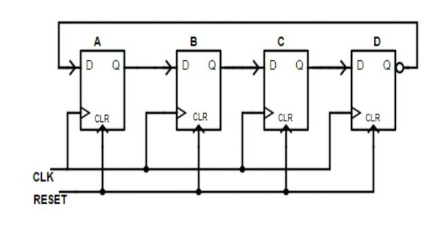Consider the 4-bit Johnson counter, it contains 4 D flip-flops, which is called 4-bit Johnson counter. It has preset and clear pins to initialize or start and reset the counted.

Reset pin acts as an on/off switch. So, the flip-flops can be enabled by clicking the Reset switch.

CLK pin is used to observe the changes in the output of the flip-flops.

Standard 2,3 and 4 stages johnson counters are used to divide the frequency of clock signals with the help of varying feedback connections. For example, a 3-stage johnson counter can be used as a 3-phase and 120 degrees phase shift square wave generator. 5-stage Johnson counter is used as a synchronous decade counter (CD4017) or divider circuit. 2-stage acts as a quadrature oscillator or generator that produces individual output signals of 90 degrees each concerning the input signal.

Truth Table

Consider the truth table of the 3-bit Johnson counter. The output of the proceeding flip-flop is connected as the input of the next flip-flop. The clock signal(CLK) is used to know the changes in the output. It contains 3 flip-flops, Q0, Q1, Q2 are the outputs of the flip-flops. The counter counts the state of cycles in a continuous closed loop.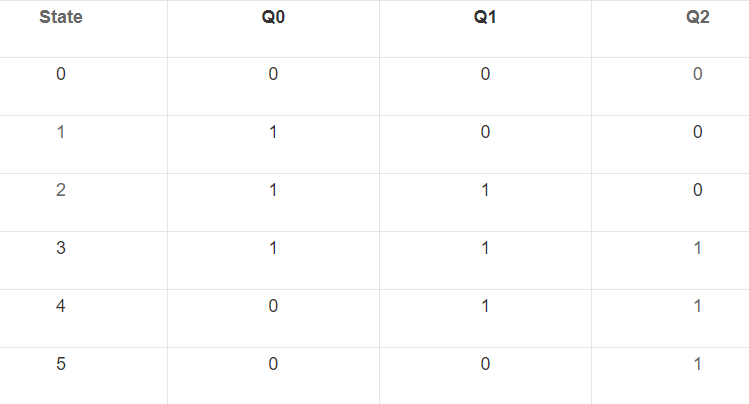# Design Synchronous BCD Counter

A synchronous BCD counter is a counter that counts from 0 to 9 in binary-coded decimal (BCD) format. It can be designed using flip-flops and combinational logic.

The circuit diagram for a synchronous BCD counter using J-K flip-flops is shown below: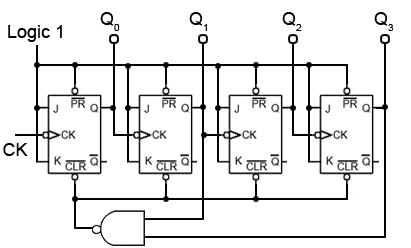The counter consists of four J-K flip-flops (FF0, FF1, FF2, and FF3) and a combinational logic circuit that generates the next state. Each flip-flop represents a decimal digit in the BCD code.

The J-K flip-flops are connected such that the output of each flip-flop is connected to the clock input of the next flip-flop. The clock input of the first flip-flop (FF0) is connected to the input clock signal.

The combinational logic circuit consists of a series of AND gates and an OR gate. The inputs to the AND gates are the outputs of the flip-flops and the complemented outputs of the flip-flops. The outputs of the AND gates are connected to the inputs of the OR gate. The output of the OR gate is used as the next state input to the flip-flops.

The truth table for the combinational logic circuit is shown below:

Inputs | Outputs

————————

Q3 Q2 Q1 Q0 | Q3+ Q2+ Q1+ Q0+

————————

0 0 0 0 | 0 0 0 1

0 0 0 1 | 0 0 1 0

0 0 1 0 | 0 0 1 1

0 0 1 1 | 0 1 0 0

0 1 0 0 | 0 1 0 1

0 1 0 1 | 0 1 1 0

0 1 1 0 | 0 1 1 1

0 1 1 1 | 1 0 0 0

1 0 0 0 | 1 0 0 1

The circuit works as follows:

1. At the beginning, all flip-flop outputs are set to 0.
2. When the clock input is high, the combinational logic circuit generates the next state based on the current state of the flip-flops.
3. The next state is then loaded into the flip-flops on the rising edge of the clock signal.
4. The counter then counts up from 0 to 9 in BCD format, and then resets to 0 and starts over again.

Note that this is a 4-bit synchronous BCD counter, so it counts from 0 to 9. For larger counters, more flip-flops and additional logic would be needed.

# Design 3-bit Synchronous Up-Counter

A 3-bit synchronous up-counter can be designed using J-K flip-flops and combinational logic. The counter counts from 0 to 7 in binary (000 to 111) and then resets to 0 and starts over again.

The circuit diagram for a 3-bit synchronous up-counter using J-K flip-flops is shown below: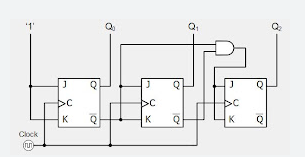The counter consists of three J-K flip-flops (FF0, FF1, and FF2) and a combinational logic circuit that generates the next state. Each flip-flop represents a bit in the binary count.

The J-K flip-flops are connected such that the output of each flip-flop is connected to the clock input of the next flip-flop. The clock input of the first flip-flop (FF0) is connected to the input clock signal.

The combinational logic circuit consists of a series of AND gates and an OR gate. The inputs to the AND gates are the outputs of the flip-flops and the complemented outputs of the flip-flops. The outputs of the AND gates are connected to the inputs of the OR gate. The output of the OR gate is used as the next state input to the flip-flops.

The truth table for the combinational logic circuit is shown below:
Inputs | Outputs

————————

Q2 Q1 Q0 | Q2+ Q1+ Q0+

————————

0 0 0 | 0 0 1

0 0 1 | 0 1 0

0 1 0 | 0 1 1

0 1 1 | 1 0 0

1 0 0 | 1 0 1

1 0 1 | 1 1 0

1 1 0 | 1 1 1

1 1 1 | 0 0 0

The circuit works as follows:

1. At the beginning, all flip-flop outputs are set to 0.
2. When the clock input is high, the combinational logic circuit generates the next state based on the current state of the flip-flops.
3. The next state is then loaded into the flip-flops on the rising edge of the clock signal.
4. The counter then counts up from 0 to 7 in binary, and then resets to 0 and starts over again.

Note that this is a 3-bit synchronous up-counter, so it counts from 0 to 7. For larger counters, more flip-flops and additional logic would be needed.

# Design 3-bit Synchronous Down-Counter

A 3-bit synchronous down-counter can be designed using J-K flip-flops and combinational logic. The counter counts from 7 to 0 in binary (111 to 000) and then resets to 7 and starts over again.

The circuit diagram for a 3-bit synchronous down-counter using J-K flip-flops is shown below: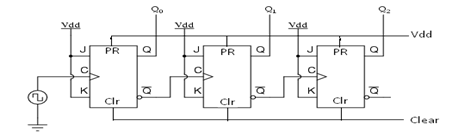The counter consists of three J-K flip-flops (FF0, FF1, and FF2) and a combinational logic circuit that generates the next state. Each flip-flop represents a bit in the binary count.

The J-K flip-flops are connected such that the output of each flip-flop is connected to the clock input of the next flip-flop. The clock input of the first flip-flop (FF0) is connected to the input clock signal.

The combinational logic circuit consists of a series of AND gates and an OR gate. The inputs to the AND gates are the outputs of the flip-flops and the complemented outputs of the flip-flops. The outputs of the AND gates are connected to the inputs of the OR gate. The output of the OR gate is used as the next state input to the flip-flops.

The truth table for the combinational logic circuit is shown below:

Inputs | Outputs

————————

Q2 Q1 Q0 | Q2- Q1- Q0-

————————

1 1 1 | 1 1 0

1 1 0 | 1 0 1

1 0 1 | 1 0 0

1 0 0 | 0 1 1

0 1 1 | 0 1 0

0 1 0 | 0 0 1

0 0 1 | 0 0 0

0 0 0 | 1 1 1

The circuit works as follows:

1. At the beginning, all flip-flop outputs are set to 1.
2. When the clock input is high, the combinational logic circuit generates the next state based on the current state of the flip-flops.
3. The next state is then loaded into the flip-flops on the rising edge of the clock signal.
4. The counter then counts down from 7 to 0 in binary, and then resets to 7 and starts over again.

Note that this is a 3-bit synchronous down-counter, so it counts from 7 to 0. For larger counters, more flip-flops and additional logic would be needed.

# Design 3-bit Synchronous Up/Down Counter

A 3-bit synchronous up/down counter can be designed using J-K flip-flops and combinational logic. The counter can count both up and down, depending on the state of the control input. The circuit diagram for a 3-bit synchronous up/down counter using J-K flip-flops is shown below: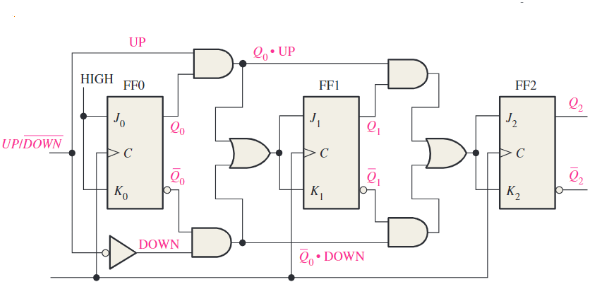The counter consists of three J-K flip-flops (FF0, FF1, and FF2), a control input (UP/DN), and a combinational logic circuit that generates the next state. Each flip-flop represents a bit in the binary count.

The J-K flip-flops are connected such that the output of each flip-flop is connected to the clock input of the next flip-flop. The clock input of the first flip-flop (FF0) is connected to the input clock signal.

The control input (UP/DN) is connected to the input of an inverter. The output of the inverter is connected to one input of an AND gate, with the other input connected to the clock input of the flip-flops.

The combinational logic circuit consists of a series of AND gates and an OR gate. The inputs to the AND gates are the outputs of the flip-flops and the complemented outputs of the flip-flops, as well as the control input and the complemented control input. The outputs of the AND gates are connected to the inputs of the OR gate. The output of the OR gate is used as the next state input to the flip-flops.

The truth table for the combinational logic circuit is shown below:

Inputs | Outputs

————————————

UP/DN Q2 Q1 Q0 | Q2+ Q1+ Q0+ Q2- Q1- Q0-

————————————

0 1 1 1 | 0 0 0 1 1 0

0 1 1 0 | 0 0 1 1 0 1

0 1 0 1 | 0 0 1 1 0 0

0 1 0 0 | 0 1 0 0 1 1

0 0 1 1 | 0 1 0 0 1 0

0 0 1 0 | 0 1 1 0 0 1

0 0 0 1 | 0 1 1 0 0 0

0 0 0 0 | 1 1 1 0 1 1

1 1 1 1 | 1 1 1 0 0 0

1 1 1 0 | 1 1 0 0 1 1

1 1 0 1 | 1 1 0 0 1 0

1 1 0 0 | 1 0 1 1 0 1

# Design MOD-6 Synchronous Counter

1. A MOD-6 synchronous counter can be designed using a 3-bit binary counter with a combinational circuit that resets the counter to zero after the count of 6.
2. The truth table for the MOD-6 counter is as follows: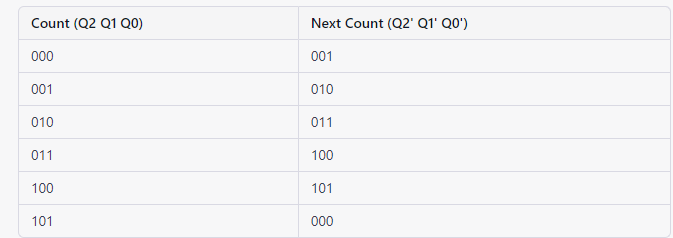Using the truth table, we can design the combinational circuit that generates the next count based on the current count.

The circuit for the MOD-6 synchronous counter using a 3-bit binary counter is shown below:

To design a MOD-6 synchronous counter using a 3-bit binary counter, we need to create a circuit that counts from 0 to 5 (MOD-6). The 3-bit binary counter can be constructed using flip-flops, and additional logic gates can be used to implement the MOD-6 behavior.

To design a MOD-6 synchronous counter using D flip-flops, we need a circuit that counts from 0 to 5 (MOD-6). Each D flip-flop will represent one bit of the counter.

Here’s the circuit diagram for a MOD-6 synchronous counter using D flip-flops: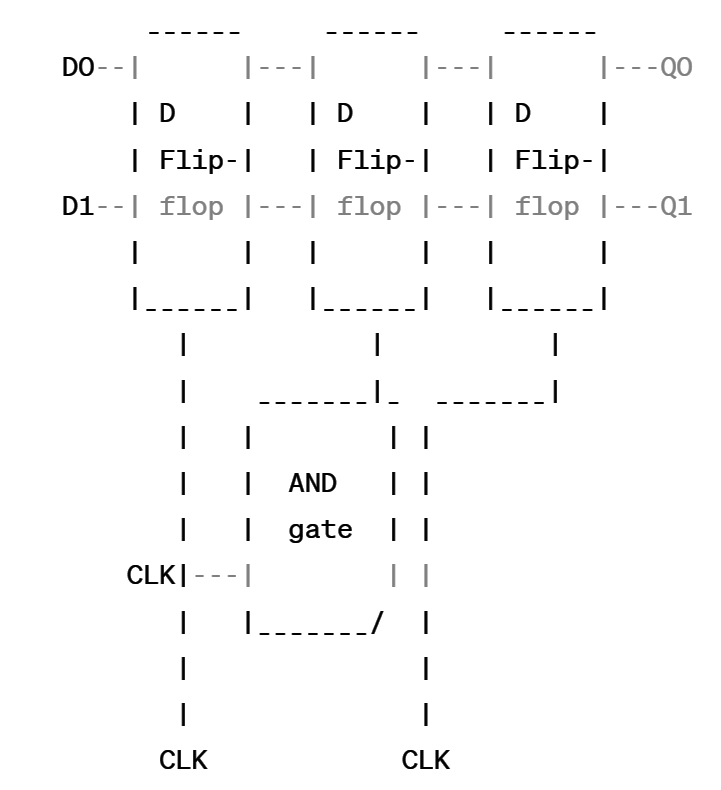In this circuit:

• Q0 and Q1 represent the outputs of the D flip-flops (Q0 is the least significant bit, and Q1 is the most significant bit).
• D0 and D1 represent the inputs of the D flip-flops.
• The AND gates are used to generate the appropriate D inputs based on the current state of the counter and the clock signal.

Here’s the connection scheme for the AND gates:

• Connect Q0 to one input of the AND gate for D0.
• Connect Q1 to one input of the AND gate for D1.
• Connect the output of the AND gate for D0 to the D input of the first D flip-flop.
• Connect the output of the AND gate for D1 to the D input of the second D flip-flop.
• Connect the clock signal (CLK) to both D flip-flops.

To achieve the MOD-6 behavior, we need to set the D inputs of the flip-flops in such a way that they count in binary from 0 to 5 (0110 in binary).

• For D0: Connect the output Q1 to one input of the AND gate for D0 and invert it (use an inverter).
• For D1: Connect the output Q1 to one input of the AND gate for D1.

With this circuit, the counter will increment from 0 to 5 (MOD-6) and repeat the sequence. The counter will advance on each rising edge of the clock signal.

Please note that in practical implementations, appropriate additional circuitry, such as reset inputs, might be required for initialization and other considerations.

# Design MOD-9 Synchronous Counter

To design a MOD-9 synchronous counter using D flip-flops, we need a circuit that counts from 0 to 8 (MOD-9). Each D flip-flop will represent one bit of the counter.

Here’s the circuit diagram for a MOD-9 synchronous counter using D flip-flops: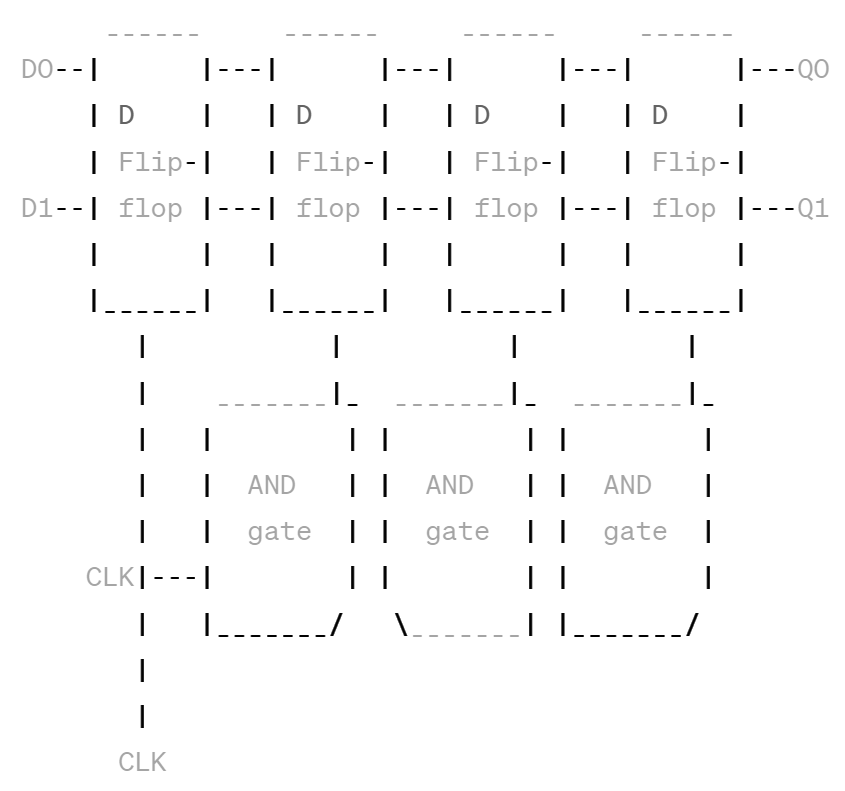In this circuit:

• Q0 and Q1 represent the outputs of the D flip-flops (Q0 is the least significant bit, and Q1 is the most significant bit).
• D0 and D1 represent the inputs of the D flip-flops.
• The AND gates are used to generate the appropriate D inputs based on the current state of the counter and the clock signal.

Here’s the connection scheme for the AND gates:

• Connect Q0 to one input of the AND gate for D0.
• Connect Q1 to one input of the AND gate for D1.
• Connect the output of the AND gate for D0 to the D input of the first D flip-flop.
• Connect the output of the AND gate for D1 to the D input of the second D flip-flop.
• Connect the clock signal (CLK) to both D flip-flops.

To achieve the MOD-9 behavior, we need to set the D inputs of the flip-flops in such a way that they count in binary from 0 to 8 (1001 in binary).

• For D0: Connect the output Q0 to one input of the AND gate for D0.
• For D1: Connect the output Q1 to one input of the AND gate for D1 and invert it (use an inverter).

With this circuit, the counter will increment from 0 to 8 (MOD-9) and repeat the sequence. The counter will advance on each rising edge of the clock signal.

Please note that in practical implementations, appropriate additional circuitry, such as reset inputs, might be required for initialization and other considerations.

# Recall Finite State Machine

A Finite State Machine (FSM) is a mathematical model used to represent a system with a finite number of states and transitions between these states based on input signals. It is a way of modelling systems that have a sequence of events or behaviours that can be described by a set of states and a set of rules that determine how the system moves from one state to another.

An FSM consists of a set of states, a set of inputs, a set of outputs, and a set of transitions between states. The current state of the FSM represents the current condition of the system, while the input signals determine the next state of the system. Each transition is associated with a condition or set of conditions that must be met in order to trigger the transition. When a transition is triggered, the FSM moves to a new state and may produce an output.

FSMs are commonly used in digital systems design to model and control the behaviour of circuits, protocols, and software applications. They can be implemented using hardware, software, or a combination of both. FSMs are also used in other fields, such as control systems, natural language processing, and artificial intelligence.

# Describe: 1. Moore Machine 2. Mealy Machine

1. Moore Machine:

A Moore machine is a type of Finite State Machine (FSM) where the output is determined by the current state of the machine only, and not by the input. In other words, the output of the machine depends only on the state that the machine is in. Moore machines are named after Edward F. Moore, who first described them in 1956.

In a Moore machine, each state has an associated output value that is produced whenever the machine is in that state. The output value is the same for every input signal that causes the machine to transition to that state. The transition function of a Moore machine determines the next state of the machine based on the current state and the input signal.

1. Mealy Machine:

A Mealy machine is another type of Finite State Machine (FSM) where the output is determined by both the current state of the machine and the input. In other words, the output of the machine depends on the state that the machine is in as well as the input signal that caused the transition. Mealy machines are named after George H. Mealy, who first described them in 1955.

In a Mealy machine, the output value is produced when the machine transitions from one state to another. The output value depends on both the current state and the input signal that caused the transition. The transition function of a Mealy machine determines the next state of the machine and the output value based on the current state and the input signal.

Here’s a tabular comparison between Moore machines and Mealy machines in digital electronics:

 Moore Machine Mealy Machine Output Dependency Outputs depend only on the current state. Outputs depend on both the current state and the inputs. Output Timing Outputs change synchronously with state transitions. Outputs can change asynchronously with input changes. State Table Contains information about the next state and outputs. Contains information about the next state and transition conditions. Input Dependency Transitions depend only on the inputs. Transitions can depend on both the inputs and the current state. Number of States Moore machines tend to have more states compared to Mealy machines. Mealy machines may have fewer states compared to Moore machines. Complexity Moore machines can be simpler to design and analyze. Mealy machines can have more complex designs and behavior. Sensitivity to Glitches Moore machines are less sensitive to input glitches as output changes are synchronized with state transitions. Mealy machines can be more sensitive to input glitches as output changes can occur immediately with input changes. Applications Suitable for applications where the output depends primarily on the current state. Suitable for applications where the output depends on both the current state and the inputs.

Both Moore and Mealy machines are used in digital systems design to model and control the behaviour of circuits, protocols, and software applications. The choice of machine type depends on the specific requirements of the system being modelled. Moore machines are simpler and easier to design, but may not be able to model complex systems that require more complex output behaviour. Mealy machines, on the other hand, are more flexible and can model more complex systems, but may be more difficult to design and implement.

# Describe Moore Machine

In digital electronics, a Moore machine is a type of finite state machine (FSM) that is named after its creator, Edward F. Moore. It is a sequential logic circuit whose outputs depend only on the current state of the machine. The Moore machine is defined by its states, inputs, outputs, and the transitions between states.

Here are the key characteristics and components of a Moore machine:

1. States: A Moore machine has a finite number of states that it can be in. These states represent different conditions or configurations of the machine.
2. Inputs: The Moore machine receives inputs from the environment or other external sources. These inputs are used to determine the transitions between states.
3. Outputs: Each state of the Moore machine is associated with a specific output value. The output remains constant throughout the duration of that state. The output is typically based on the current state and does not depend on the inputs directly.
4. Transitions: The transitions between states occur based on specific conditions and the inputs received. Each state has one or more conditions that must be met for a transition to occur. These conditions are typically represented by logical expressions involving the inputs.
5. State Diagram: A Moore machine can be represented graphically using a state diagram. The state diagram shows the states as nodes and the transitions between states as directed edges. Each edge is labeled with the condition that triggers the transition.

The behavior of a Moore machine is defined by the combination of its states, inputs, outputs, and transitions. The outputs of a Moore machine are associated with the current state and are independent of the inputs. The outputs are updated only when a state transition occurs.

Moore machines are commonly used in various applications, including control systems, digital circuits, and sequential logic design. They are particularly useful in situations where the output depends solely on the state and does not require immediate responsiveness to input changes.

To design a Moore machine, the following steps are typically followed:

1. Identify the problem or desired behavior that the machine needs to achieve.
2. Define the states based on the problem requirements.
3. Determine the inputs that affect the state transitions.
4. Specify the outputs associated with each state.
5. Define the transition conditions that cause state changes.
6. Construct the state diagram to visualize the states and transitions.
7. Implement the Moore machine using digital logic gates and flip-flops.

Overall, Moore machines provide a systematic and structured approach to designing sequential logic circuits, allowing for the implementation of complex behaviors and functionalities in digital systems.

# Describe Mealy Machine

In digital electronics, a Mealy machine is a type of finite state machine (FSM) named after its creator, George H. Mealy. It is a sequential logic circuit that produces outputs based on both the current state of the machine and the inputs it receives. The Mealy machine is defined by its states, inputs, outputs, and the transitions between states.

Here are the key characteristics and components of a Mealy machine:

1. States: A Mealy machine has a finite number of states that it can be in. These states represent different conditions or configurations of the machine.
2. Inputs: The Mealy machine receives inputs from the environment or other external sources. These inputs are used to determine the transitions between states and affect the output values.
3. Outputs: The outputs of a Mealy machine are associated with both the current state and the inputs. The output values can change based on the combination of the current state and the inputs.
4. Transitions: The transitions between states occur based on specific conditions and the inputs received. Each state has one or more conditions that must be met for a transition to occur. These conditions are typically represented by logical expressions involving the inputs.
5. Output Functions: Mealy machines have output functions associated with the transitions between states. These functions define the output values produced when a particular transition occurs. The output functions are often represented as logical expressions involving the current state and the inputs.
6. State Diagram: A Mealy machine can be represented graphically using a state diagram. The state diagram shows the states as nodes and the transitions between states as directed edges. Each edge is labeled with the condition that triggers the transition and the corresponding output value.

The behavior of a Mealy machine is defined by the combination of its states, inputs, outputs, transitions, and output functions. The outputs of a Mealy machine depend on both the current state and the inputs received. The outputs can change immediately with the input changes, as they are not solely dependent on the current state.

Mealy machines are commonly used in various applications, including control systems, digital circuits, and sequential logic design. They are particularly useful in situations where the output depends on both the state and the inputs, allowing for more flexibility in output generation.

To design a Mealy machine, the following steps are typically followed:

1. Identify the problem or desired behavior that the machine needs to achieve.
2. Define the states based on the problem requirements.
3. Determine the inputs that affect the state transitions and output generation.
4. Specify the outputs associated with each state-transition combination.
5. Define the transition conditions that cause state changes.
6. Construct the state diagram to visualize the states, transitions, and output functions.
7. Implement the Mealy machine using digital logic gates and flip-flops.

Overall, Mealy machines provide a versatile approach to designing sequential logic circuits, allowing for outputs that are dependent on both the current state and the inputs. They offer a powerful tool for modeling and implementing complex behaviors and functionalities in digital systems.

# Describe Minimization of Sequential Machine

To illustrate the minimization of a sequential machine using a state table, let’s consider an example with a state table that describes the behavior of a sequential machine.

State Table Example:

Suppose we have a sequential machine with four states (A, B, C, D), two inputs (X, Y), and one output (Z). The state table represents the next state and output for each combination of current state and inputs.

 Current State Input (X, Y) Next State Output (Z) A 0 0 A 0 A 0 1 B 1 A 1 0 C 0 A 1 1 D 1 B 0 0 A 1 B 0 1 B 0 B 1 0 C 1 B 1 1 D 0 C 0 0 A 0 C 0 1 B 1 C 1 0 C 0 C 1 1 D 1 D 0 0 A 1 D 0 1 B 0 D 1 0 C 1 D 1 1 D 0

To minimize the sequential machine, we can follow these steps:

Step 1: Grouping Equivalent States

• Identify any states that have the same outputs for the same inputs and can transition to the same next states.
• In our example, states B and C have the same output and can transition to the same next states for all input combinations (0 0, 0 1, 1 0, 1 1). Thus, we can consider B and C as equivalent states.

Step 2: Create the Reduced State Table

• Replace the equivalent states with a single representative state.
• Update the next state and output columns accordingly.
• In our example, we can merge B and C into a single state, BC. The reduced state table becomes:
 Current State Input (X, Y) Next State Output (Z) A 0 0 A 0 A 0 1 BC 1 A 1 0 BC 0 A 1 1 D 1 BC 0 0 A 0 BC 0 1 BC 1 BC 1 0 BC 0 BC 1 1 D 1 D 0 0 A 1 D 0 1 BC 0 D 1 0 BC 1 D 1 1 D 0

Step 3: Verify the Minimized Sequential Machine

• Check if the minimized sequential machine behaves the same as the original one.
• Simulate different input sequences on both the original and minimized sequential machines to compare the outputs and next state transitions. Ensure they match.

By minimizing the sequential machine, we have reduced the number of states required to achieve the same functionality. This can lead to a more efficient and simplified design, with potential benefits in terms of reduced complexity, power consumption, and circuit size.

# Recall State Reduction Methods: Successive Partitioning

Successive partitioning is a state reduction method used to minimise a Finite State Machine (FSM) by iteratively partitioning the set of states into groups of equivalent states. The method starts with an initial partition of the states, such as a partition into two groups of even and odd numbered states, and then refines this partition until the minimum number of states is achieved.

The successive partitioning method involves several iterations, where in each iteration, the partition is refined by merging groups of states that are equivalent. The equivalence of states is determined by comparing their next-state functions and output functions. If two states produce the same next-state functions and output functions for all inputs, they are considered equivalent and can be merged into a single group.

The successive partitioning method is a simple and effective way to minimise a sequential machine, but it may not always produce the optimal solution. The method can be computationally expensive for large FSMs and may require multiple iterations to converge to a minimal solution. Other state reduction methods, such as the Hopcroft-Karp algorithm, can be more efficient and provide better results for large FSMs.

# Recall State Reduction Methods: Implication Chart

The Implication Chart is a state reduction method used to minimise a Finite State Machine (FSM) by identifying and eliminating redundant states. The method involves constructing an implication chart that shows the dependencies between the states in the machine and then iteratively eliminating redundant states until the minimal number of states is achieved.

To construct an implication chart, the next-state functions of the machine are compared for all pairs of states. If the next-state functions of state A imply the next-state functions of state B for all inputs, then A is said to imply B and an implication arrow is drawn from A to B in the chart.

The implication chart is then used to identify and eliminate redundant states in the machine. A state is considered redundant if it can be reached by two or more different paths in the chart. Redundant states are then merged into a single state, and the implication chart is updated to reflect the new dependencies between the states.

The Implication Chart method is a powerful technique for minimising a sequential machine, but it can be computationally expensive for large FSMs and may require multiple iterations to converge to a minimal solution. Other state reduction methods, such as the Hopcroft-Karp algorithm, can be more efficient and provide better results for large FSMs.

# Describe Method of State Assignment

The method of state assignment is a technique used in digital system design to assign binary codes to the states of a Finite State Machine (FSM) after it has been minimised. The purpose of state assignment is to optimise the design of the FSM by reducing its cost, improving its performance, and minimising its power consumption.

The method of state assignment involves assigning unique binary codes to each state of the minimised FSM. There are several criteria that can be used to assign the binary codes, such as:

1. One-hot encoding: In this method, each state is assigned a unique code consisting of a single bit set to ‘1’ and all other bits set to ‘0’. This method is simple and efficient but requires a large number of flip-flops, which can increase the cost of the design.
2. Grey encoding: In this method, adjacent states are assigned binary codes that differ by only one bit. This method reduces the number of transitions between the states and can improve the performance of the design.
3. Minimum transition encoding: In this method, the binary codes are assigned to minimise the number of transitions between the states. This method can reduce the power consumption of the design by reducing the number of transitions.

The method of state assignment is an important step in digital system design because it can significantly impact the performance, cost, and power consumption of the design. The choice of state assignment method depends on the specific requirements of the design and the trade-offs between cost, performance, and power consumption.

# Recall Hazards and Its types

In digital circuits, a hazard is a temporary glitch or error that can occur in the output of a logic function due to a delay in the propagation of signals through the circuit. Hazards can cause incorrect or unexpected behaviour in the circuit and can be classified into the following types:

1. Static Hazard: A static hazard occurs when a change in the input signal causes a temporary change in the output signal, even though the input signal has not completed its transition. This can happen when there are multiple paths between the input and the output of the circuit, and the delays along the paths are not perfectly matched.
2. Dynamic Hazard: A dynamic hazard occurs when a change in the input signal causes the output signal to momentarily switch to an incorrect value before settling to the correct value. This can happen when the input signal changes while the circuit is transitioning from one state to another.
3. Essential Hazard: An essential hazard is a type of dynamic hazard that cannot be eliminated by adding more gates or by optimising the circuit design. It is caused by the inherent logic function of the circuit and can only be eliminated by changing the logic function.

Hazards can be eliminated or reduced by adding delay elements, such as buffers or inverters, to the circuit or by optimising the circuit design to ensure that the delays along all paths are properly matched. Hazards can also be avoided by using hazard-free logic families, such as the ECL (Emitter-Coupled Logic) and CML (Current-Mode Logic) families.

# Differentiate between Static and Dynamic Hazards

Static and dynamic hazards are two types of hazards that can occur in digital circuits. The main difference between them is the timing of the glitch or error that causes the hazard.

Static hazards occur when a change in the input signal causes a temporary change in the output signal, even though the input signal has not completed its transition. This means that the output signal may change to an incorrect value and then return to the correct value, creating a glitch or error in the output. Static hazards can occur due to delays in the circuit or when there are multiple paths between the input and output of the circuit.

On the other hand, dynamic hazards occur when a change in the input signal causes the output signal to momentarily switch to an incorrect value before settling to the correct value. This means that the output signal may oscillate or toggle between the correct and incorrect values before finally settling on the correct value. Dynamic hazards can occur due to the propagation delays in the circuit or when the input signal changes while the circuit is transitioning from one state to another.

In summary, the key difference between static and dynamic hazards is that static hazards occur due to a delay in the signal propagation, while dynamic hazards occur due to a transition in the signal values. Both types of hazards can be eliminated or reduced by adding delay elements to the circuit or by optimising the circuit design to ensure that the delays along all paths are properly matched.

# Determine Hazards in Combinational Circuits

Hazards can occur in combinational circuits when there are multiple paths between the inputs and outputs, and the delays along these paths are not properly matched. The following steps can be used to determine hazards in a combinational circuit:

1. Draw the logic diagram of the circuit and identify the critical path(s) between the inputs and outputs.
2. Determine the signal transition times for each input and output signal, based on the propagation delays of the gates along the critical path(s).
3. Identify any input patterns that could cause a glitch or error in the output signal due to differences in the signal transition times. These input patterns are called hazard-inducing patterns.
4. Check the output signals of the circuit for each of the hazard-inducing patterns. If any output signal changes to an incorrect value and then returns to the correct value, a static hazard is present. If the output signal oscillates or toggles between the correct and incorrect values, a dynamic hazard is present.
5. If hazards are present, add delay elements such as buffers or inverters to the circuit to properly match the signal delays along all paths.

Alternatively, hazards can also be avoided by using hazard-free logic families, such as the ECL (Emitter-Coupled Logic) and CML (Current-Mode Logic) families.

# Recall Faults and its types

In digital circuits, a fault is an abnormal condition that results in an incorrect signal or behaviour. There are several types of faults that can occur in digital circuits, including:

1. Stuck-at fault: This occurs when a signal is permanently stuck at a logic level, either high or low. Stuck-at faults are the most common type of fault and can be caused by a defective transistor or a broken wire.
2. Delay fault: This occurs when the delay of a signal through a gate or wire is longer or shorter than expected. Delay faults can be caused by variations in manufacturing processes or environmental conditions.
3. Bridging fault: This occurs when two or more wires are short-circuited, causing a signal to be connected to an unintended path.
4. Transition fault: This occurs when a signal transitions from one logic level to another incorrectly, such as a signal that should transition from low to high but transitions from high to low instead.
5. Coupling fault: This occurs when the changing signal in one wire affects the signal in another wire, resulting in an incorrect output.
6. Power or ground faults: These occur when a power or ground line is missing or has an open circuit, or when a power or ground line is short-circuited to another line.

Faults can be detected and diagnosed using fault simulation tools, which generate test vectors that detect faults and determine the location and type of the fault. Fault coverage measures the ability of the test vectors to detect faults in a circuit, and fault grading assigns a severity level to each fault based on its impact on the circuit’s performance.

# Differentiate between Struck-At and Bridging Faults

Stuck-at and bridging faults are two types of faults that can occur in digital circuits. The main differences between them are:

1. Stuck-at fault: This fault occurs when a signal is permanently stuck at a logic level, either high or low. It is caused by a defective transistor or a broken wire. Stuck-at faults are the most common type of fault, and they can be easily detected and diagnosed using automatic test equipment.
2. Bridging fault: This fault occurs when two or more wires are short-circuited, causing a signal to be connected to an unintended path. It is caused by a defect in the manufacturing process, such as a metal particle or a void in the oxide layer. Bridging faults are less common than stuck-at faults, and they are more difficult to detect and diagnose using automatic test equipment.

In summary, the main differences between stuck-at and bridging faults are that stuck-at faults are caused by a defective transistor or a broken wire, they are the most common type of fault, and they are easily detected and diagnosed. Bridging faults are caused by a defect in the manufacturing process, they are less common than stuck-at faults, and they are more difficult to detect and diagnose.

# Differentiate between Single Stuck-At and Multiple Stuck-At Faults

A stuck-at fault is a type of fault in which a signal line is permanently stuck at either a high or low logic level. The difference between a single stuck-at fault and multiple stuck-at faults is the number of signal lines that are affected.

In a single stuck-at fault, only one signal line is affected and stuck at a particular logic level. This can be caused by a defect in a transistor or a broken wire. The effect of a single stuck-at fault on the circuit’s behaviour is relatively easy to analyse, and the fault can be detected using automatic test equipment (ATE) by comparing the output of the circuit under test to the expected output for a given input.

In contrast, a multiple stuck-at fault occurs when more than one signal line is affected by a stuck-at fault. This can be caused by a defect in a logic gate or a wiring error. The effect of a multiple stuck-at fault on the circuit’s behaviour can be more complex and harder to analyse than a single stuck-at fault. Detecting multiple stuck-at faults can also be more challenging, as the fault may cause the circuit to produce correct outputs for some inputs while producing incorrect outputs for other inputs.

In summary, a single stuck-at fault affects only one signal line, while a multiple stuck-at fault affects more than one signal line. The effect of a multiple stuck-at fault on the circuit’s behaviour is typically more complex than a single stuck-at fault, and detecting and diagnosing multiple stuck-at faults can be more challenging.

# Describe Faults Detection in Combinational and Sequential circuits

Fault detection is the process of identifying and locating faults or errors in digital circuits. There are various techniques used for fault detection in combinational and sequential circuits.

For combinational circuits, the most common method of fault detection is the use of automatic test pattern generation (ATPG) techniques. In this method, a set of test patterns is generated to detect faults in the circuit. These test patterns are applied to the circuit, and the output response is compared to the expected output response. Any discrepancies between the actual and expected outputs indicate a fault in the circuit. The ATPG process can be used to detect single or multiple stuck-at faults, as well as other types of faults such as bridging faults.

For sequential circuits, fault detection is more complex than for combinational circuits. One method of fault detection in sequential circuits is to use fault simulation. In this method, a fault is simulated in the circuit, and the circuit’s response is analysed to identify the fault. Sequential circuit fault simulation requires the use of test vectors that represent the sequence of inputs applied to the circuit. The output response of the circuit is then compared to the expected output response.

Another method of fault detection in sequential circuits is to use built-in self-test (BIST) techniques. In this method, a special test circuit is added to the original circuit to generate test patterns and detect faults. The BIST circuit generates test patterns and applies them to the circuit, and the circuit’s response is analysed to detect faults.

In summary, fault detection in digital circuits is a critical step in ensuring the correct operation of the circuit. The methods used for fault detection depend on the type of circuit, and the most common techniques include ATPG, fault simulation, and BIST.